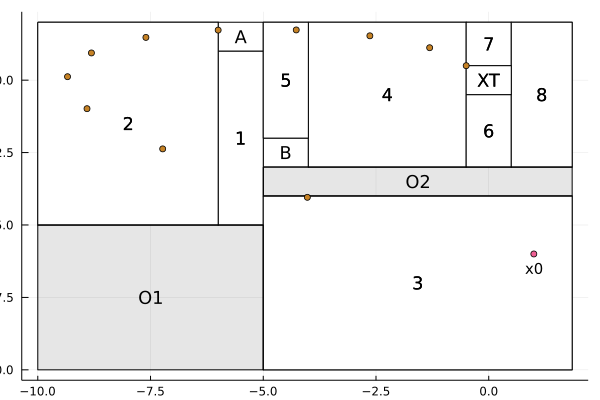# Example: Gol, Lazar and Belta (2013)This example reproduces parts of the numerical results of . A similar example reproducing all results of  is available as a codeocean capsule in .

This example was borrowed from [1, Example VIII.A] and tackles an optimal control for the hybrid system with state evolution governed by

$$$x(k+1) = \begin{bmatrix} 1 & 1 \\ 0 & 1 \end{bmatrix}x(k) + \begin{bmatrix} 0.5 \\ 1.0 \end{bmatrix} u(k)$$$

The goal is to take the state vector toward a target set XT by visiting one of the squares A or B and avoiding the obstacles O1 and O2

First, let us import CDDLib, GLPK, OSQP, JuMP, Pavito and Ipopt

import CDDLib
import GLPK
import OSQP
using JuMP
import Pavito
import Cbc
import Ipopt

At this point we import Dionysos

using Dionysos

And the file defining the hybrid system for this problem

include(joinpath(dirname(dirname(pathof(Dionysos))), "examples", "gol_lazar_belta.jl"))
gol_lazar_belta (generic function with 1 method)

Now we instantiate our system using the function provided by gol_lazar_belta.jl

system = gol_lazar_belta(CDDLib.Library(), Float64);

Then, we define initial conditions (continuous and discrete states) to this system and set N as the search depth, i.e., the number of allowed time steps.

x0 = [1.0, -6.0];
q0 = 3;

N = 11;

We instantiate our Optimal Control Problem by defining the state and transition costs. Notice that Comment that state_cost is defined to be zero for each mode/discrete state of the system and the transition_cost is defined to be u_1^2 which is defined by the quadratic form u' * Q * u with Q = ones(1, 1).

state_cost = Fill(ZeroFunction(), nmodes(system))

problem = OptimalControlProblem(
system,
q0, x0,
Fill(state_cost, N),
Fill(Fill(transition_cost, ntransitions(system)), N),
system.ext[:q_T],
N
);

Notice that we used Fill for all N time steps as we consider time-invariant costs. Finally, we select the method presented in  as our optimizer

qp_solver = optimizer_with_attributes(
OSQP.Optimizer,
"eps_abs" => 1e-8,
"eps_rel" => 1e-8,
"max_iter" => 100000,
MOI.Silent() => true
);

mip_solver = optimizer_with_attributes(
Cbc.Optimizer,
MOI.Silent() => true
);

cont_solver = optimizer_with_attributes(
Ipopt.Optimizer,
MOI.Silent() => true
);

miqp_solver = optimizer_with_attributes(
Pavito.Optimizer,
"mip_solver" => mip_solver,
"cont_solver" => cont_solver,
MOI.Silent() => true
);

"continuous_solver" => qp_solver,
"mixed_integer_solver" => miqp_solver,
"indicator" => false,
"log_level" => 0
);

and use it to solve the given problem, with the help of the abstraction layer MathOptInterface provided by JuMP

optimizer = MOI.instantiate(algo)
MOI.set(optimizer, MOI.RawParameter("problem"), problem)
MOI.optimize!(optimizer)
┌ Warning: Infeasible NLP problem terminated with primal status: NEARLY_FEASIBLE_POINT. This cannot be as this NLP problem was feasible by design.
└ @ Pavito ~/.julia/packages/Pavito/H744k/src/algorithm.jl:428

We check the solver time

MOI.get(optimizer, MOI.SolveTime())
2.3714439868927

the termination status

termination = MOI.get(optimizer, MOI.TerminationStatus())
OPTIMAL::TerminationStatusCode = 1

the objective value

objective_value = MOI.get(optimizer, MOI.ObjectiveValue())
11.385062952226304

and recover the corresponding continuous trajectory

xu = MOI.get(optimizer, ContinuousTrajectoryAttribute());

A little bit of data visualization now:

using Plots
using Colors

##Auxiliary function for annotating
function text_in_set_plot!(pl, po, t; kws...)
##solve finding center (other solvers? https://jump.dev/JuMP.jl/dev/installation/#Supported-solvers)
solver = optimizer_with_attributes(GLPK.Optimizer, "presolve" => GLPK.ON)
plot!(pl, po; kws...)
if t !== nothing
c, r = hchebyshevcenter(hrep(po), solver, verbose=0)
annotate!(pl, [(c..., text(t, 12))])
end
end

##Initialize our canvas
p = Plots.plot(fmt = :png, fillcolor = :white)

##Show the discrete modes
for mode in states(system)
t = (system.ext[:q_T] in [mode, mode + 11]) ? "XT" : (mode == system.ext[:q_A] ? "A" : (mode == system.ext[:q_B] ? "B" :
mode <= 11 ? string(mode) : string(mode - 11)))
text_in_set_plot!(p, stateset(system, mode), t, fillcolor = :white, linecolor = :black)
end

##Plot obstacles
for i in eachindex(system.ext[:obstacles])
text_in_set_plot!(p, system.ext[:obstacles][i], "O\$i", fillcolor = :black, fillalpha = 0.1)
end

##Initial state
scatter!(p, [x0], [x0])
annotate!(p, [(x0, x0 - 0.5, text("x0", 10))])

##Split the vector into x1 and x2
x1 = [xu.x[j] for j in eachindex(xu.x)]
x2 = [xu.x[j] for j in eachindex(xu.x)]

##Plot the trajectory
scatter!(p, x1, x2)1. Gol, E. A., Lazar, M., & Belta, C. (2013). Language-guided controller synthesis for linear systems. IEEE Transactions on Automatic Control, 59(5), 1163-1176.
2. Bemporad, A., & Morari, M. (1999). Control of systems integrating logic, dynamics, and constraints. Automatica, 35(3), 407-427.
3. Legat B., Bouchat J., Jungers R. M. (2021). Abstraction-based branch and bound approach to Q-learning for hybrid optimal control. 3rd Annual Learning for Dynamics & Control Conference, 2021.
4. Legat B., Bouchat J., Jungers R. M. (2021). Abstraction-based branch and bound approach to Q-learning for hybrid optimal control. https://www.codeocean.com/. https://doi.org/10.24433/CO.6650697.v1.